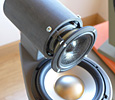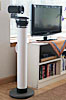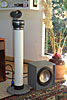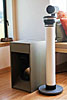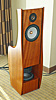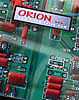----- Experience in your own room the magical nature of stereo sound -----

 Resources Sound recordings Links Other designs My current setup About me Site map

 ------------------ Digital Photo Processes

 ------------------ The Sea Ranch

 ------------------ My Daughter the Jeweler

# Crossovers

--- Crossovers --- Linkwitz/Riley --- Active filters ---

# Crossovers

 The best crossovers ... The best electrical crossover filter is one that maintains the acoustic polar response of a loudspeaker throughout the crossover frequency range as output shifts from one driver to the next. The sum of acoustic lowpass and highpass outputs must have allpass behavior without high Q peaks in the group delay. The highpass filter section must attenuate out-of-band driver terminal voltages at a sufficiently high rate so that cone excursion decreases with decreasing frequency and nonlinear distortion is minimized. The crossover must be inaudible The crossover must be inaudible on program material. This also implies that the power response of the two drivers must be similar in the crossover region, and that requires special attention during the loudspeaker's concept and design phases. Crossovers may be implemented either as passive RLC networks, as active filters with operational amplifier circuits or with DSP engines and software. The only excuse for passive crossovers is their low cost. Their behavior changes with the signal level dependent dynamics of the drivers. They block the power amplifier from taking maximum control over the voice coil motion. They are a waste of time, if accuracy of reproduction is the goal. Accurate Stereo performance tests                                                                                       SL - October 2009

It is practically impossible for a single radiator to cover the 20 Hz to 20 kHz audio frequency range at adequate sound pressure levels (SPL) and with wide dispersion off-axis. At a 50 Hz bass frequency, for example, it takes an air volume displacement of 214 cm3 to generate 90 dB SPL at 1 m distance from the source in free space. Take a typical 6.5 inch (132 mm effective) diameter driver and its cone would have to move 14 mm peak-to-peak linearly, which by far exceeds its excursion capability (Ref. 4).
At 1000 Hz, though, the same driver would have to vibrate only with (50/1000)2 = 1/400th of this excursion for the same 90 dB SPL, but now the cone is in its breakup region and no longer acts as a rigid piston, causing energy storage and deteriorating off-axis radiation. Thus, this driver will have to be complemented with a larger one for low frequencies, and a smaller one to extend the highs, in order to obtain full frequency range coverage. The electrical audio signal then needs to be divided between three drivers.

The proper crossover of signals from one driver to the next is a subject of debate amongst audiophiles with some preferring a1st order Butterworth filter function . As often done, a single capacitor and resistor in the connection to the tweeter will not necessarily create a phase-linear 6 dB/octave crossover, but more something like a 18 dB/oct. acoustic response due to the inherent 12 dB/oct. highpass behavior of the tweeter itself. Furthermore, the tweeter will contribute a good amount of intermodulation distortion because the cone excursion wants to rise at 12 dB/oct. towards lower frequencies for constant SPL and the driver is not built for that. Add to this the wide frequency coverage overlap between tweeter and midrange, with the resulting irregular polar radiation pattern, and the 6 dB/oct. crossover becomes a costly solution for achieving waveform fidelity. At best, the goal may be obtained over a very small region in space and over a limited frequency range. But, does it matter to the listening experience, given some common observations?

Networks that divide the electrical audio signal between different drivers must be designed with the driver characteristics and the driver layout in mind in order to obtain the desired acoustic crossover function and polar radiation pattern in conjunction with tolerable non-linear and linear distortion of the acoustic output. All crossovers involve design tradeoffs. For the Audio Artistry Beethoven-Elite system, for example, I used 24 dB/oct. and 12 dB/oct. Linkwitz-Riley (Ref. 17) and 6 dB/oct. Butterworth acoustic crossover filter responses. My latest design, the ORION, employs two 24 dB/oct filters for highest accuracy of perceived sound.

I have a strong preference for line level active dividing networks ahead of the power amplifiers (Ref. 2, 12, 17). In this approach the power amplifier output is connected directly - except for a very low resistance speaker cable - to the voice coil of the driver. The amplifier takes maximum control over the motion of the speaker cone which gives a greater sense of clarity and dynamism compared to a passive dividing network between amplifier and driver. Active crossovers make much more effective use of amplifier power. A clipping woofer amplifier is not seen by the tweeter, which has its own amplifier. The clipping of the woofer amplifier may not even be noticed in this case. It would surely be heard with a passive crossover, where it might even overheat and damage the tweeter, because of the large amount of high frequency energy in the clipped signal.

Crossover filters for a speaker usually incorporate frequency response corrections for the individual drivers to obtain a desired overall response. The active network has the advantage of correcting easily for different sensitivities of drivers and equalizing not only the individual drivers but the combined response as well. Not having to deal with the interaction between driver impedance and passive filter network gives the designer of an active crossover/equalizer much greater freedom and control to develop a superior product.

Crossover topology mistakes

------------------------------------------------------

### The Duelund 3-way crossover filter function D3

Recently I received an intriguing paper of the late Steen Duelund from one of his admiring followers. Steen, a "Danish Speaker Maniac", gives a fun derivation of known crossover filter functions for the mathematically inclined and he found some novel ones. To follow his text more easily I rewrite some of it here and develop it further to show a potentially useful 3-way allpass crossover filter.

A) Transient-perfect crossovers

1) - First order crossover with 6 dB/oct slopes for highpass and lowpass filters = Butterworth, B1+

H1(s) = (s + 1) / (s + 1) = 1  where s = s +jw and the pole at sp = -1 and zero at sz = -1 cancel each other
H1 = [s / (s + 1)] + [1 / (s + 1)] = HP + LP ==> 2-way

2) - Second order crossover by squaring H1(s) ==> Baekgaard

(H1)2 = (s2 + 2s + 1) / (s + 1)2
(H1)2 = [s2 / (s + 1)2] + [2s / (s + 1)2] + [1 / (s +1)2] = HP + BP + LP ==> 3-way  

Highpass and lowpass filters have 12 dB/oct slopes, but the filler bandpass filter has only 6 dB/oct slopes and is difficult to realize. At the crossover frequencies w1 = 0.5 and w2 = 2 the three outputs are not in phase and the axis of the vertical polar pattern will tilt when the three separate drivers are aligned vertically. This can be avoided by using two filler drivers in a W-F-T-F-W arrangement instead of W-F-T.

More practical solutions of transient-perfect crossovers are based on delay derived crossovers where  HP(s) = e-sT - LP(s)   or they are constructed from lowpass and highpass filters which overlap in the crossover region in such a way that a minimum phase response is preserved . Their summed response is not flat, but since it is minimum phase it can be equalized. Steeper slopes can be obtained from these two types of crossovers, but the lack of in-phase addition in the crossover region tends to require symmetrical driver arrangements.

For further study I recommend:
 E. Baekgaard, " A novel approach to linear-phase loudspeakers using passive crossover networks"
JAES, Vol. 25, pp. 284-294, May 1977
 S. Lipshitz & J. Vanderkooy, "A family of linear-phase crossover networks of high slope derived by time delay", JAES, Vol. 31, pp. 2-19, January/February 1983
 S. Lipshitz & J. Vanderkooy, "Use of frequency overlap and equalization to produce high-slope linear-phase loudspeaker crossover networks", JAES, Vol. 33, pp. 114-126, March 1985

B) Allpass crossovers

3) - First order crossover with 6 dB/oct slopes for highpass and lowpass filters = Butterworth, B1-

H2(s) = (s - 1) / (s + 1) = 1ejf   when s = jw,  i.e. the magnitude is one, but there is phase shift F(w)

The pole at sp = -1 and zero at sz = +1 do not cancel but form a 1st order allpass.

4) - 2nd order Linkwitz-Riley filter (12 dB/oct, LR2)

H2 H1 = (s - 1) (s + 1) / (s +1) (s + 1) = (1 - s2) / (s + 1)2
H2 H1 = [1 / (s + 1)2] - [s2 / (s + 1)2] = LP - HP  ==> 2-way and 1st order allpass as above.

HP and LP are 2 x 90 degrees out of phase at all frequencies and the polarity of one driver must be reversed to obtain allpass behavior.

5) - Squaring H2(s) and going to a more general expression by replacing '2' with 'a'

(H2)2 = (s2 - 2s + 1) / (s2 + 2s +1)
H3 = (s2 - as + 1) / (s2 + as +1)

6) - 4th order Linkwitz-Riley filter (24 dB/oct, LR4) by squaring H3

(H3)2 = (s2 - as +1)2 / (s2 + as +1)2 = [s4 - s2(a2 - 2) + 1] / (s2 + as + 1)2

Let a = sqrt(2) = 1.41, then

H4 = (s4 + 1) / (s2 + 1.41s + 1)2  ==> 2-way
which is a 2nd order allpass with a complex pole pair at  -0.71 +/- j0.71
and a pair of complex zeros at +0.71 +/- j0.71 in the right half s-plane.
The individual filter functions are:

HP4 = s4 / (s2 + 1.41s + 1)  and     LP4 = 1 / (s2 + 1.41s + 1)

HP and LP are 4 x 90 degrees out of phase at all frequencies, i.e. they are in-phase.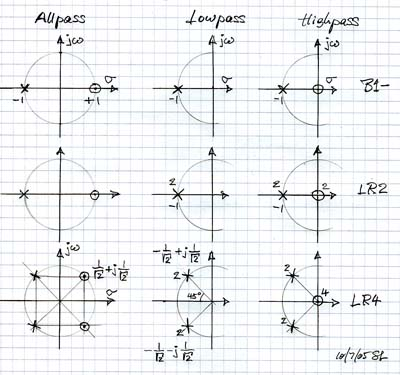7) - Let a = 4 in (H3)2 to obtain a 3-way allpass crossover

H5 = (s2 - 4s +1)2 / (s2 + 4s +1)2  ==> 3-way

This is an allpass with two real axis poles at -3.73 and two at -0.27. It has real axis zeros at +/-3.73 and +/-0.27 which combine to two 1st order allpasses.

The numerator of the polynomial is  [s4 -s2(a2 - 2) + 1] = s4 - 14s2 + 1
The individual filter functions are:

HP5 = s4 / (s2 + 4s +1)
It is 4th order, but has in practice only 12 dB/oct filter slopes because the poles are widely spread apart.

BP5 = -14s2 / (s2 + 4s +1)2
The bandpass filter slopes are 12 dB/oct.

LP5 = 1 / (s2 + 4s +1)2
Practical filter slopes are only 12 dB/oct

Crossover is at -6 dB points and the drivers are in phase so there is no tilt in the polar response. The filter slopes should be steeper though to make the crossover more realizable.8) - Squaring (H3)2 once more leads to (H3)4 and selecting a = 3 gives the allpass

H6 = (s2 - 3s +1)4 / (s2 + 3s +1)4

There are 8 poles as calculated from (s2 + 3s +1)4  = 0, four at sp1 = -2.62 and four at sp2 = -0.38

Expanding the numerator yields (s8 - 14s6 + 51s4 - 14s2 + 1) and thus the numerator for the bandpass function becomes

NBP = -14s2 [s4 - (51/14)s2 +1]   with two zeros each at sz1 = -1.83, sz2 = -0.55 and sz3 = 0

The individual filter functions are:

LP6 = 1 / [(s + 2.62)4 (s +0.38)4]
The lowpass is 8th order, but has in practice only 24 dB/oct filter slopes because the poles are widely spread apart.

HP6 = s8 / [(s + 2.62)4 (s +0.38)4]
Highpass filter slopes are 24 dB/oct as above.

BP6 = -14s2 (s + 1.83)2 (s + 0.55)2 / [(s + 2.62)4 (s +0.38)4]
The bandpass filter slopes are 12 dB/oct. The filter is centered at
wc = 1 and the crossover frequencies are w1 = 0.24 and w2 = 4.1 at -6 dB as read from the graph. Thus a 3-way loudspeaker with 120 Hz and 2.05 kHz crossover frequencies could be built that uses these filter functions. The gentle slopes of the bandpass filter function impose stringent requirements upon the midrange driver's volume displacement capability and its frequency range in order to obtain the targeted acoustic response.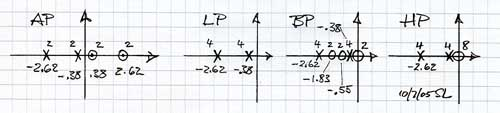9) - It is interesting to compare this Duelund 3-way filter function to a crossover that uses LR4 filters. The crossover frequencies must be at w1 = 0.24 and w2 = 4.1 and thus the LR4 poles are located at
0.24*(-0.707 +/- j0.707) and at 4.1*(-0.707 +/- j0.707)

The filter functions become

LPLR4 = 0.244 / (s2 + 0.34s + 0.242)2

BPLR4 = 4.14 s4 / [(s2 + 0.34s + 0.242)2 (s2 + 5.8s + 4.12)2]

HPLR4 = s4 / (s2 + 5.8s + 4.12)2

The sum of the three filters is approximately allpass since the two crossover frequencies are far (1:17) apart.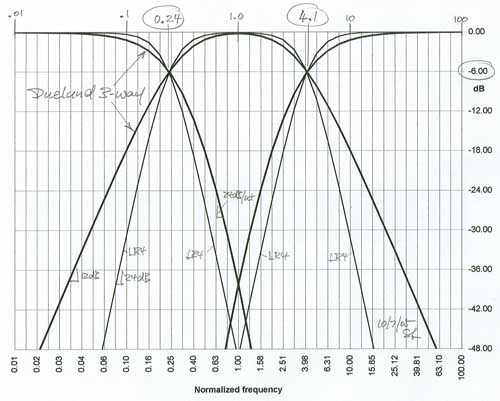The Duelund filters roll off more gradually through the crossover region though highpass and lowpass reach 24 dB/oct slope. The bandpass covers a wider frequency range due to its more gradual 12 dB/oct roll-off. The group delay of the 3-way also reflects this relative behavior. In particular the Duelund does not show any peaking because the allpass filter sections are 1st order squared. Based on my experimentation with 1st and 2nd order allpass crossovers this visually smoother response has no audible benefits since the amount of peaking is very small.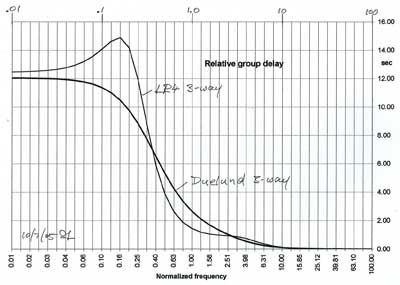The frequency response curves and the group delay were determined in a spreadsheet using the methodology shown in 12db-hpf.gif.

10) The Duelund 3-way is based on an 8th order polynomial yet the maximum slopes are only 4th order over the first 40 dB of attenuation. It seems likely that the same response can be approximated with 4th order functions. The good match, which was arrived at by an educated guess, can be seen in the graph below.In the s-plane representation one can see the dominant poles taking over and cancellations of several adjacent pole-zero pairs.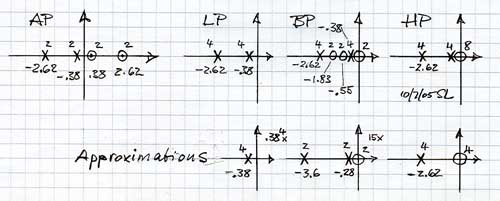Thus a practical Duelund 3-way crossover would have parameters like this:

 a) Crossover frequencies at 0.24 and 4.1 (e.g. at 120 Hz and 2050 Hz) Crossover points are at -6 dB. Filter outputs are in phase when the midrange bandpass has reversed polarity. Bandpass is centered at normalized frequency 1.0 (e.g. at 500 Hz). b) Woofer lowpass  LPD3 = 0.384 / ( s + 0.38)4  Four real axis poles at -0.38 which can be realized with two LR2 lowpass filters in cascade (e.g. at 190 Hz). c) Midrange bandpass  BPD3 = -15s2 / [(s + 0.28)2 (s + 3.6)2] Two zeros at the origin and two poles at -0.28 can be realized with a single LR2 highpass filter (e.g. at 140 Hz).  The two poles at -3.6 can be realized with one LR2 lowpass (e.g. at 1800 Hz). It is in cascade with the LR2 highpass. d) Tweeter highpass  HPD3 = s4 / (s + 2.62)4 Four zeros at the origin and four poles at -2.62 can be realized with two LR2 highpass filters in cascade (e.g. at 1310 Hz).

Note that all mathematical functions are meant to describe the acoustic response of a 3-way loudspeaker. To the extend that woofer, midrange or tweeter drivers have a non-flat frequency response they must be either equalized to be flat, or their response must be made part of the filter function. In particular, the midrange driver's natural low frequency roll-off could be equalized with a "Linkwitz Transform" to obtain the desired LR2 highpass behavior of c) above. The tweeter can be treated similarly for two of the poles of d). The woofer's natural highpass behavior causes a phase lead which is probably far from zero at the first crossover frequency and thus affects the proper addition of woofer and midrange outputs. This can be corrected by placing a 1st order allpass in the midrange channel which simulates the highpass phase shift of the woofer.

The Duelund 3-way crossover could provide a useful option for building a loudspeaker.What you hear is not the air pressure variation in itself  but what has drawn your attention in the streams of superimposed air pressure variations  at your eardrums An acoustic event has dimensions of Time, Tone, Loudness and Space Have they been recorded and rendered sensibly? ___________________________________________________________ Last revised: 11/25/2020   -  © 1999-2019 LINKWITZ LAB, All Rights Reserved